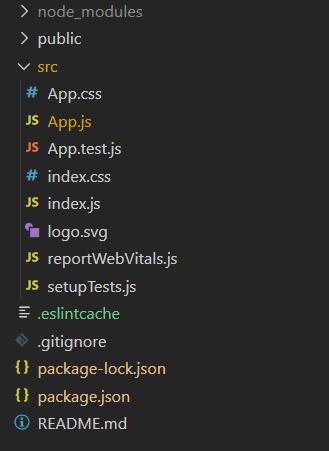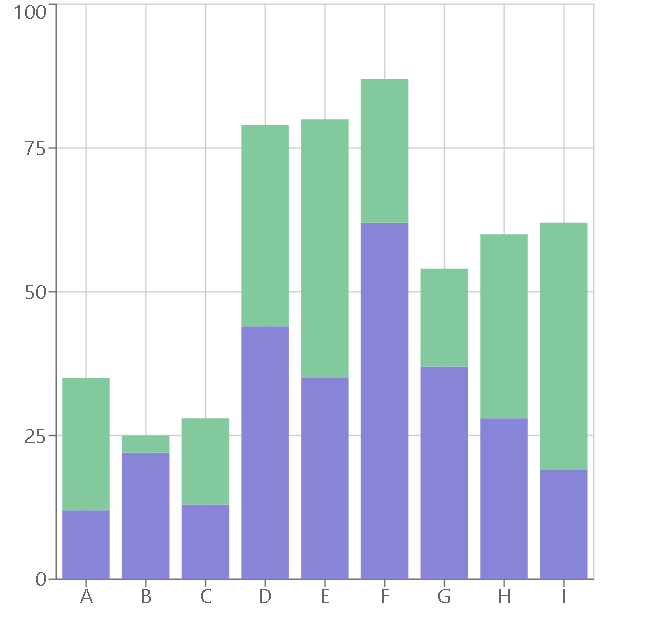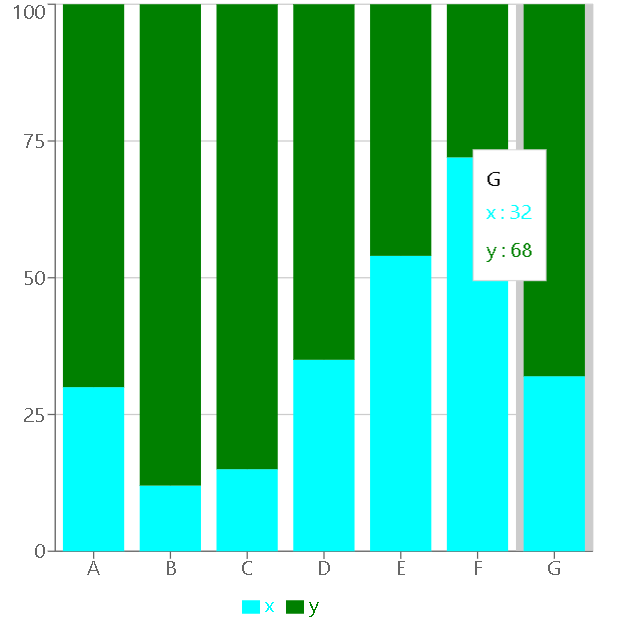Related Articles

# Create a Stacked Bar Chart using Recharts in ReactJS

• Difficulty Level : Expert
• Last Updated : 30 Jul, 2021

Introduction: Rechart JS is a library that is used for creating charts for React JS. This library is used for building Line charts, Bar charts, Pie charts, etc, with the help of React and D3 (Data-Driven Documents).

A stacked Bar Chart is the extension of a basic bar chart. It displays various discrete data in the same bar chart for a better comparison of data.

Approach: To create a Stacked Bar Chart we use the BarChart component of recharts npm package. We firstly create a cartesian grid and X-axis and Y-Axis. Then add multiple Bar charts using Bar component and to get them stacked on top of each other use the same stackId for all charts.

Creating React Application And Installing Module:

• Step 1: Create a React application using the following command.

`npx create-react-app foldername`
• Step 2: After creating your project folder i.e. foldername, move to it using the following command.

`cd foldername`
• Step 3: After creating the ReactJS application, Install the required modules using the following command.

`npm install --save recharts`

Project Structure: It will look like the following.Example 1: In this example, we will create a basic stacked bar chart using BarChart and Bar component of recharts npm package. To stack the two bars on top of each other we will add same stackId to both Bar components. Now write down the following code in the App.js file. Here, App is our default component where we have written our code.

## App.js

 `import React from ``'react'``;``import { BarChart, Bar, XAxis, YAxis, ``    ``CartesianGrid } from ``'recharts'``;`` ` `const App = () => {`` ` `    ``// Sample data``    ``const data = [``        ``{ name: ``'A'``, x: 12, y: 23, z: 122 },``        ``{ name: ``'B'``, x: 22, y: 3, z: 73 },``        ``{ name: ``'C'``, x: 13, y: 15, z: 32 },``        ``{ name: ``'D'``, x: 44, y: 35, z: 23 },``        ``{ name: ``'E'``, x: 35, y: 45, z: 20 },``        ``{ name: ``'F'``, x: 62, y: 25, z: 29 },``        ``{ name: ``'G'``, x: 37, y: 17, z: 61 },``        ``{ name: ``'H'``, x: 28, y: 32, z: 45 },``        ``{ name: ``'I'``, x: 19, y: 43, z: 93 },``    ``];`` ` `    ``return` `(``        ````            ````            ````            ````            ````            ````        ````    ``);``}`` ` `export ``default` `App;`

Step to Run Application: Run the application using the following command from the root directory of the project:

`npm start`

Output: Now open your browser and go to http://localhost:3000/, you will see the following output:Output

Example 2: In this example, we will change the color of bars using fill property. To add a tooltip that will display information about bar on hover and legend that will show labels for stacked bars, we will use Tooltip component and Legend component. Now change the following code in the App.js file.

## App.js

 `import React from ``'react'``;``import { BarChart, Bar, XAxis, YAxis, CartesianGrid,``        ``Legend, Tooltip } from ``'recharts'``;`` ` `const App = () => {`` ` `    ``// Sample data``    ``const data = [``        ``{ name: ``"A"``, x: 30, y: 70 },``        ``{ name: ``"B"``, x: 12, y: 88 },``        ``{ name: ``"C"``, x: 15, y: 85 },``        ``{ name: ``"D"``, x: 35, y: 65 },``        ``{ name: ``"E"``, x: 54, y: 46 },``        ``{ name: ``"F"``, x: 72, y: 28 },``        ``{ name: ``"G"``, x: 32, y: 68 }``    ``];`` ` `    ``return` `(``        ````            ````            ````            ````            ````            ````            ````            ````        ````    ``);``}`` ` `export ``default` `App;`

Output: Save the project using CTRL+S.Now open your browser and go to http://localhost:3000/, you will see the following output:Output

Hey geek! The constant emerging technologies in the world of web development always keeps the excitement for this subject through the roof. But before you tackle the big projects, we suggest you start by learning the basics. Kickstart your web development journey by learning JS concepts with our JavaScript Course. Now at it’s lowest price ever!

My Personal Notes arrow_drop_up